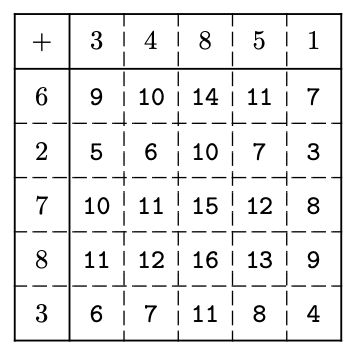시간 제한 메모리 제한 제출 정답 맞은 사람 정답 비율
1 초 512 MB 7 7 7 100.000%

## 문제

Pathfinding is a task of finding a route between two points. It often appears in many problems. For example, in a GPS navigation software where a driver can query for a suggested route, or in a robot motion planning where it should find a valid sequence of movements to do some tasks, or in a simple maze solver where it should find a valid path from one point to another point. This problem is related to solving a maze.

The maze considered in this problem is in the form of a matrix of integers A of N × N. The value of each cell is generated from a given array R and C of N integers each. Specifically, the value on the ith row and jth column, cell (i, j), is equal to Ri + Cj. Note that all indexes in this problem are from 1 to N.

A path in this maze is defined as a sequence of cells (r1, c1),(r2, c2), . . . ,(rk, ck) such that |ri − ri+1| + |ci − ci+1| = 1 for all 1 ≤ i < k. In other words, each adjacent cell differs only by 1 row or only by 1 column. An even path in this maze is defined as a path in which all the cells in the path contain only even numbers.

Given a tuple <ra, ca, rb, cb> as a query, your task is to determine whether there exists an even path from cell (ra, ca) to cell (rb, cb). To simplify the problem, it is guaranteed that both cell (ra, ca) and cell (rb, cb) contain even numbers. For example, let N = 5, R = {6, 2, 7, 8, 3}, and C = {3, 4, 8, 5, 1}. The following figure depicts the matrix A of 5 × 5 which is generated from the given array R and C.Let us consider several queries:

• <2, 2, 1, 3>: There is an even path from cell (2, 2) to cell (1, 3), e.g., (2, 2),(2, 3),(1, 3). Of course, (2, 2),(1, 2),(1, 3) is also a valid even path.
• <4, 2, 4, 3>: There is an even path from cell (4, 2) to cell (4, 3), namely (4, 2),(4, 3).
• <5, 1, 3, 4>: There is no even path from cell (5, 1) to cell (3, 4). Observe that the only two neighboring cells of (5, 1) are cell (5, 2) and cell (4, 1), and both of them contain odd numbers (7 and 11, respectively), thus, there cannot be any even path originating from cell (5, 1).

## 입력

Input begins with a line containing two integers: N Q (2 ≤ N ≤ 100 000; 1 ≤ Q ≤ 100 000) representing the size of the maze and the number of queries, respectively. The next line contains N integers: Ri (0 ≤ Ri ≤ 106) representing the array R. The next line contains N integers: Ci (0 ≤ Ci ≤ 106) representing the array C. The next Q lines each contains four integers: ra ca rb cb (1 ≤ ra, ca, rb, cb ≤ N) representing a query of <ra, ca, rb, cb>. It is guaranteed that (ra, ca) and (rb, cb) are two different cells in the maze and both of them contain even numbers.

## 출력

For each query in the same order as input, output in a line a string “YES” (without quotes) or “NO” (without quotes) whether there exists an even path from cell (ra, ca) to cell (rb, cb).

## 예제 입력 1

5 3
6 2 7 8 3
3 4 8 5 1
2 2 1 3
4 2 4 3
5 1 3 4


## 예제 출력 1

YES
YES
NO


## 예제 입력 2

3 2
30 40 49
15 20 25
2 2 3 3
1 2 2 2


## 예제 출력 2

NO
YES